# Short run cost

Short run cost is such a cost which is incurred during the short run of a production process. We know that in the short run there are some factors which are fixed, while others are variable. Similarly, short run costs are also divided into two kinds of costs – Fixed Costs and Variable Costs. Let us look at the different short run costs in detail.

### Short Run Total Cost (TC)

The sum total of the fixed cost and the variable cost is known as the Total Cost (TC). There are two types of total costs, Total Fixed Cost (TFC) and Total Variable Cost (TVC). Total cost is the total expenditure incurred by a firm on the factors of production required for the production of a commodity. TC is the sum of TFC and TVC at various levels of output.

Total Fixed Cost (TFC) refers to those short run costs which do not directly vary with the level of output. TFC is incurred on fixed factors like machinery, plant, land, building etc., which cannot be changed in the short run. The payment to these factors remains the same irrespective of the level of output. TFC remains the same whether the output is large, small or even zero. For example, rent of premises, interest on loan, salary of permanent staff, insurance premium.

Total Variable Costs (TVC) refers to those short run costs which directly vary with the level of output. TVC is incurred on variable factors like raw material, direct labour, power, etc., which change with the level of output. This means that TVC rises with increase in output and falls with decrease in output. TVC is incurred till there is production and it becomes zero at zero level of production.

The concept of Total Cost can be better understood with a schedule and diagram given below,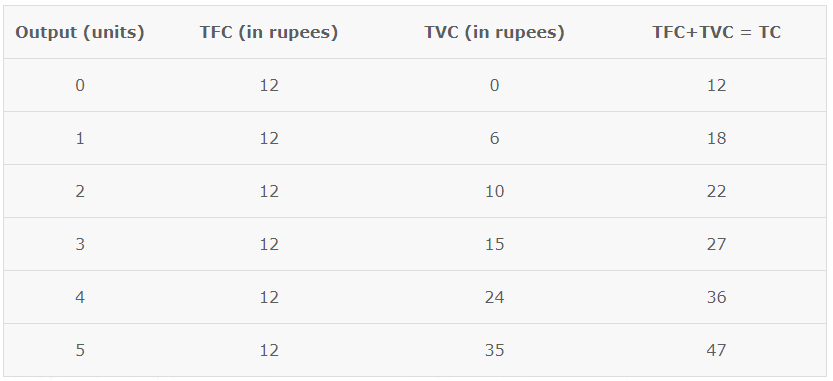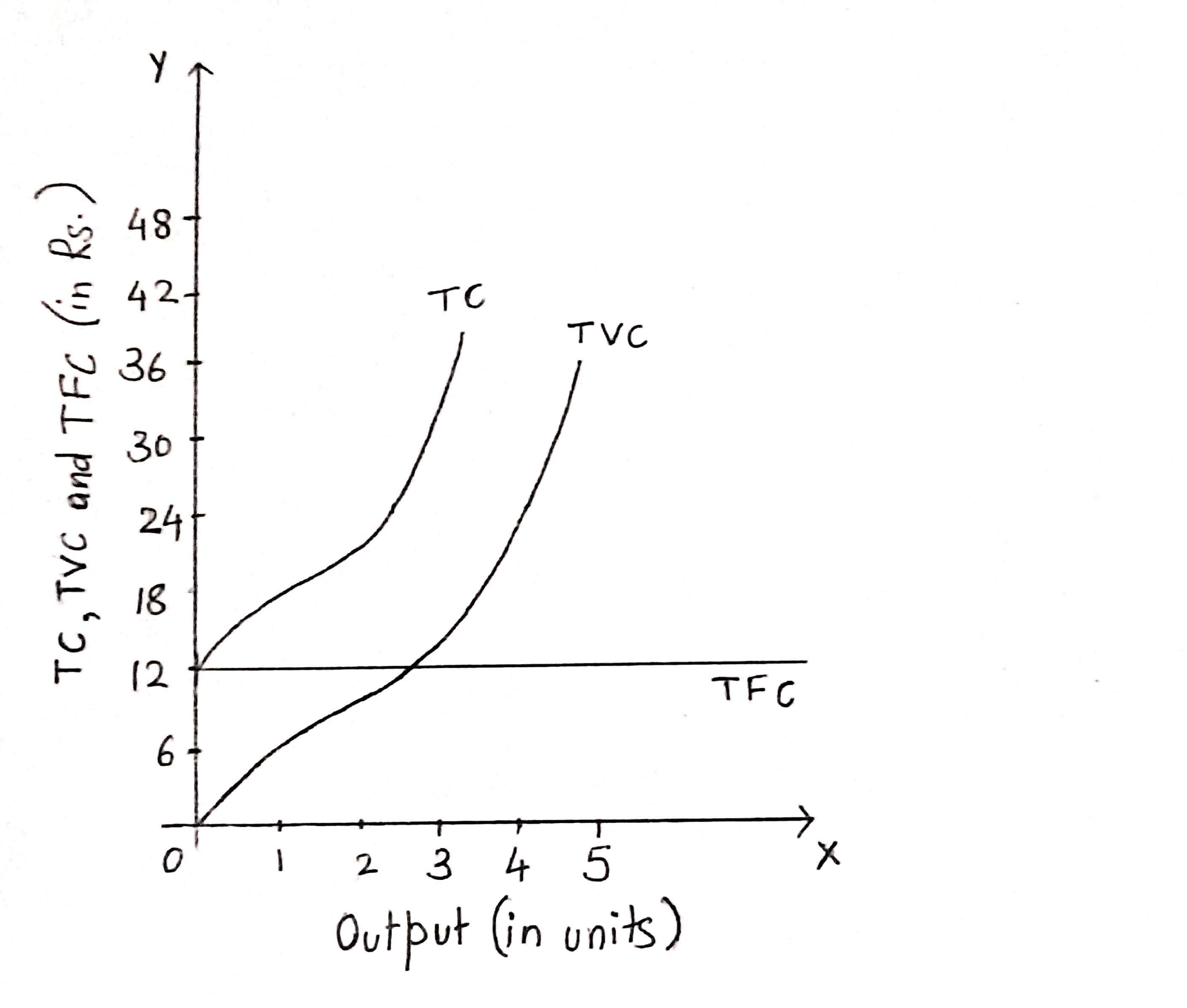We can observe the following things from the graph given above –

1. TFC curve is a horizontal straight line parallel to the x-axis as it remains constant at all levels of output.
2. TC ad TVC are inversely S-shaped because they rise initially at a decreasing rate, then at a constant rate and thn at a increasing rate. This is because of the law of variable proportions.
3. At zero output, TC is equal to TFC as there is no variable cost at zero level of output.
4. The vertical distance between the TFC curve and the TC curve is equal to TVC.
5. TC and TVC are parallel to each other and the constant vertical distance between them represent TFC.

### Short Run Marginal Cost (MC)

Marginal cost refers to those short run costs which are an addition to total cost when one more unit of output is produced.

MCn = TCn – TCn-1

Where,
MCn = Marginal cost of nth unit
TCn = Total cost of n units
TCn-1 = Total cost of (n-1) units
n = number of units of produced

We now know that MC is the change in TC when one more unit of output is produced. However, when change in units produced is more than one unit, then MC can be calculated as,

MC = Change in Total Cost/ Change in units of Output

MC = ΔTC/ΔQ

If TC of producing 2 units is Rs. 200 and TC of producing 5 units is Rs. 350, then MC will be,

MC = TC of 5 – TC of 2/5 -2

MC = 350-200/5-2 = 150/3

MC = Rs. 50

We know that MC is the change in TC when one more unit of output is produced, ad TC = TFC + TVC. TFC is not affected by change in the level of output, therefore, MC is independent of TFC and is affected only by TVC. Therefore, MC can also be written as,

MCn = TVCn – TVCn-1

Let us now understand the concept of MC with the help of a schedule and diagram,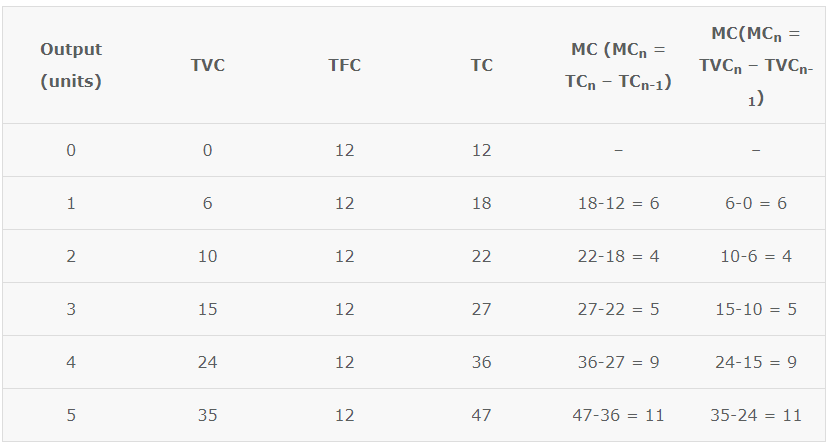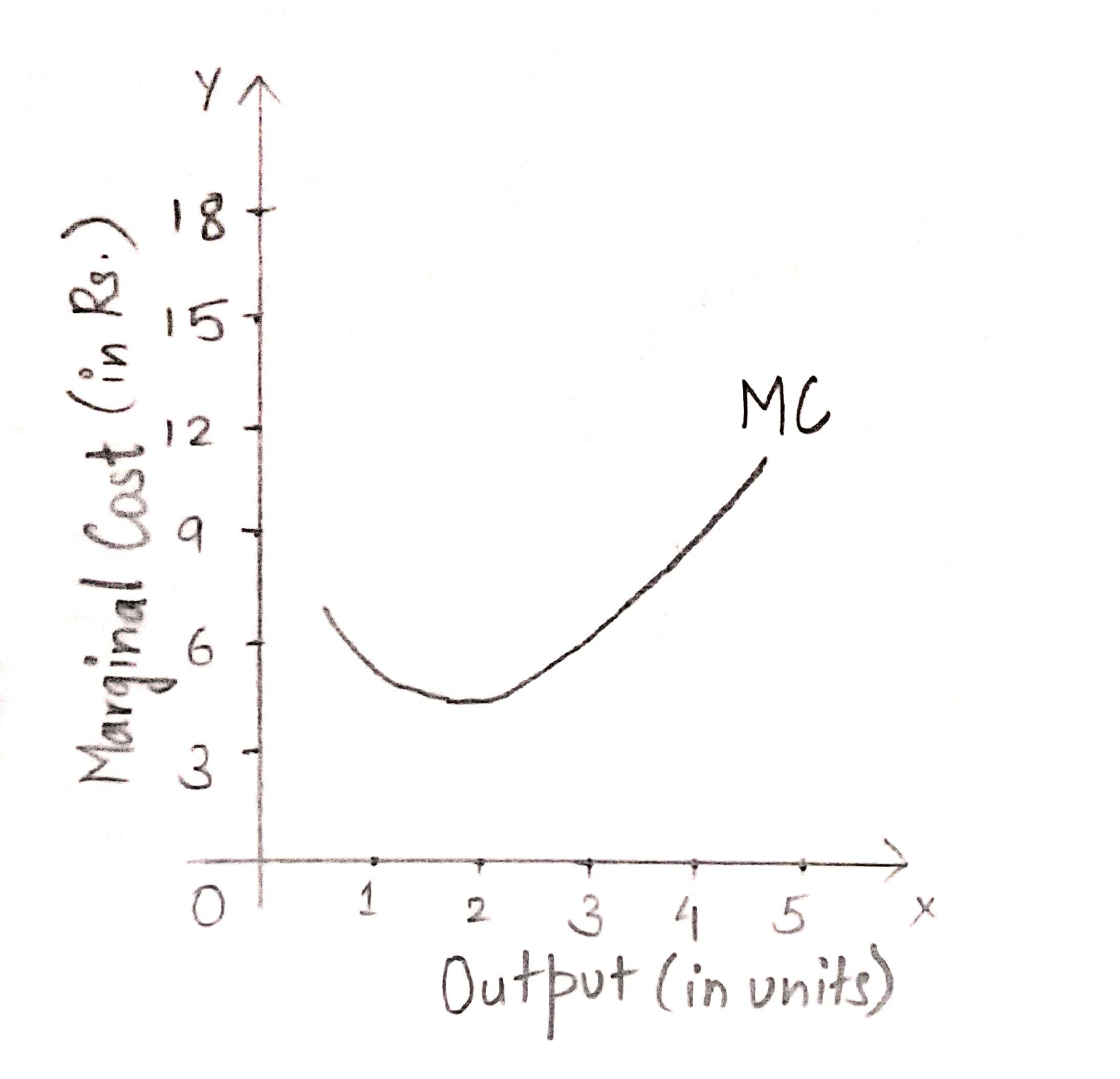MC can be calculated from both TC and TVC. The MC curve is obtained by plotting the points shown in the table. As we can see from the graph, MC curve is U-shaped, i.e. MC falls till it reaches its minimum point and thereafter it starts rising. The reason behind its U-shape is the Law of Variable Proportions.

### Short Run Average Cost (AC)

The per unit short run costs explain the relationship between cost and output in a more realistic manner. From Total Fixed Cost (TFC), Total Variable Cost (TVC) and Total Cost (TC), we can obtain Average Fixed Cost (AFC), Average Variable Cost (AVC) and Average Cost (AC) or Average Total Cost (ATC).

Average Fixed Cost (AFC) refers to the fixed cost of production per unit. It is calculated by dividing the TFC by total output (Q),

AFC = TFC/Q

AFC falls with increase in output because TFC remains the same at all levels of output in the short run.

Average Variable Cost (AVC) refers to the per unit variable short run cost of production. It is calculated by dividing TVC by total output.

AVC = TVC/Q

AVC initially falls with increase in output. Once the output rises to an optimum level, AVC starts rising.

Average Total Cost or Average Cost (AC) refers to the per unit total cost of production. It is calculated by dividing TC by total output.

AC = TC/Q

It can also b defined as the sum of AFC and AVC,

AC = AFC + AVC

We can understand all these concepts better with the help of a schedule and a graph.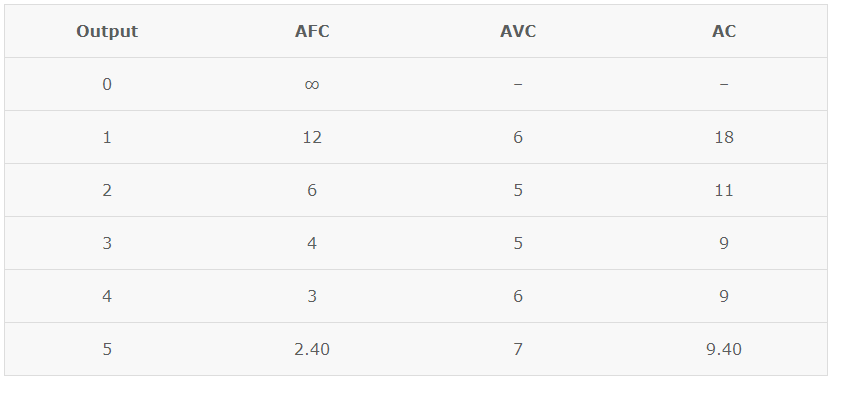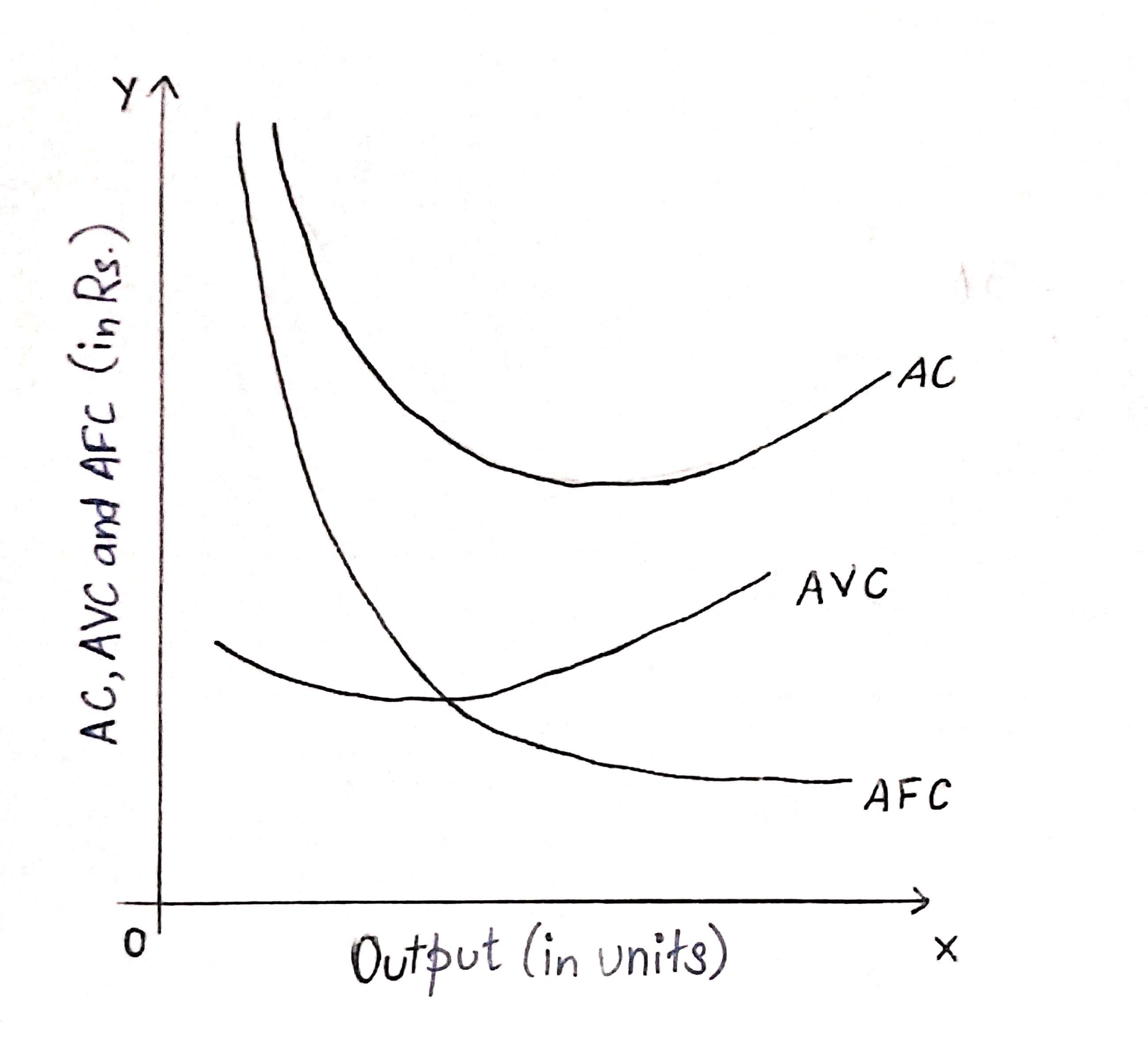As we can see from the graph given above, AC curve will always lie above the AVC curve, because AC at all levels of outputs includes both AVC and AFC. The AFC curve s a rectangular hyperbola and never touches either of the axes. As the output increases the gap between AC and AVC curves decreases but they never intersect each other. This happens because the vertical distance between them is AFC which can never be zero.